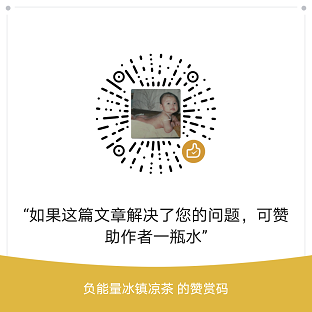﻿ sql server的批量更新和字符串关联加索引

# sql server的批量更新和字符串关联加索引

2022/3/19 17:42:01站长

```update tableA set column1=t.val
from (
select s.key,s.val
from (
values(1,2),(2,3),(3,4)
) s (key,val)
) t
where tableA.column2=t.key
-----------------------------------------
update tableA set column1=t.val
from (
select
case when c.mode=1 then b.num*100-335
else (b.num-200*1.2) end as val,
b.key
from tableB b
inner join tableC c on b.cid=c.id
) t
where tableA.column2=t.key```

`update tableA set column1=2 where key='a';`